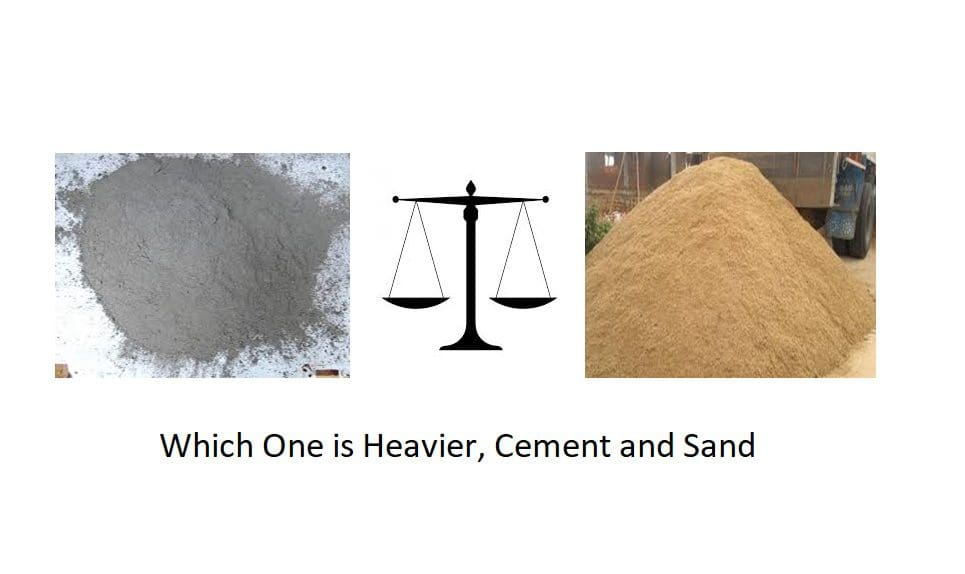# Which is One Heavier, Cement or Sand?

By weight a ton of cement and a ton of sand weigh the same amount, but by volume in an identical bags/containers of same capacity, we will get more cement (heavier) than sand i.e. 1 cubic meter of cement will be heavier to 1 cum of sand, this is because of cement mineralogical composition (composition of its raw materials) differ than that of natural sand.To clarify further, let us understand specific gravity.

The specific gravity of a material gives us how much more or less dense the material is than water (taken as standard material).

Water has a specific gravity of 1.000 (near 4°C). If a material is denser than water, then its specific gravity is greater than 1. If it is less dense than water, then the specific gravity is less than 1.

Specific gravity of sand ranges 2.6 – 2.7 and that of cement is 3.14 – 3.15, whereas diesel has specific gravity ranges 0.82 to 0.95. Since the specific gravity of diesel is less than water it floats on top of it.

As specific gravity of sand is 2.6 – 2.7 and that of cement is 3.14 – 3.15, i.e. for the same volume occupied by cement and sand, cement is “3.15/2.7 = 1.16 times “heavier than sand.

People often get confused between bulk density and specific gravity , dry bulk density of cement is 1440 kg/cum and that of natural sand is 1600 kg/cum. This does not imply that sand is heavier to cement. The right comparison would be specific gravity of each of them or any other construction material to understand which one is “Heavier”